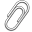[Documentation] [TitleIndex] [WordIndex]

## Overview

The different controllers are implemented in this package. The controllers have access to an actuator which contains the hand data updated in real time by sr_edc_ethercat_drivers and sr_robot_lib.

Each controller is sending force demands to a motor at 1kHz (an internal force control loop is running at 5kHz on the motor boards).

## Friction Compensation

A friction compensation algorithm is available for each controller. The idea behind the friction algorithm is very simple: due to the tendon friction, the force to apply to move a joint is a function of the current joint position and the direction which you want to take.

The sr_friction_compensation package is used to record and interpolate the friction compensation map. Based on this map, we just add a value to the output of the controller as illustrated below (we have two different maps, one for each direction):In our controllers, we're using what we call an hysteresis deadbands for avoiding to drive the motors unnecessarily: when we're entering a small deadband, if the error changes sign, we're not driving the motor, unless we leave a bigger deadband:## Controller Types

### Effort Controllers

This is a very simple controller, as the real force control loop is done on the motor board. The force demand is directly transmitted to the motor, after limiting it to a range specified in the parameters and adding the friction compensation, except in the specified deadband.

### Velocity Controllers

This is a PID controller transforming a velocity demand into a force demand. First (if we're outside of a given deadband) the velocity error is fed to a pid loop. The friction compensation is then added to the output of this loop (if we're outside of a certain deadband), and the motor demand is limited to the range specified by the user.

### Position Controllers

This is a PID controller transforming a position demand into a force demand. First (if we're outside of a given deadband) the position error is fed to a pid loop. The friction compensation is then added to the output of this loop (if we're outside of a certain deadband), and the motor demand is limited to the range specified by the user.

### Mixed Position / Velocity Controllers

This controller is a position controller on top of a velocity controller. This controller ensures that the joint travels at a given speed, and slows down to stop on the target.

Based on the position error, a velocity demand is generated from this graph (the minimum / maximum velocity as well as the slope can be modified):The velocity demand is then fed to the velocity controller described previously, which generates a force demand to send to the motor.

2022-11-26 13:05